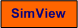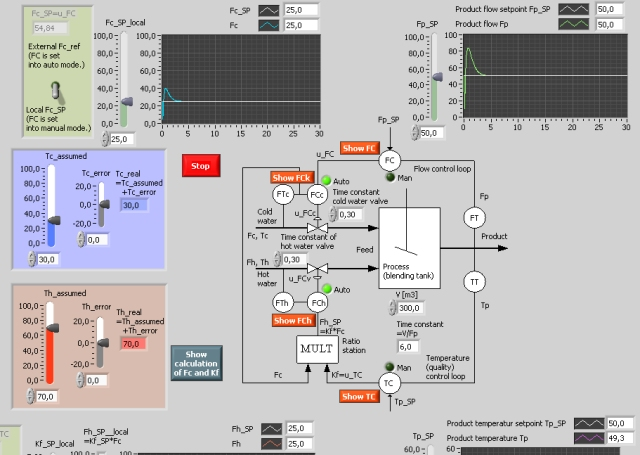# Control of Product Quality and Flow Incuding Ratio Control

Snapshot of the front panel of the simulator:• What is needed to run the the simulator? Read to get most recent information!
• Tips for using the simulator.
• The simulator: . The simulator runs immediately after the download by clicking Open in the download window. Alternatively, you can first save a copy of the exe-file on any directory (folder) on your PC and then run the exe-file, which starts the simulator.

## Description of the simulated system

A process in the form of a tank blending cold and hot water is simulated. The product flow Fp and the product quality, which is here assumed to be the product temperature Tp, are controlled by adjusting or manipulating two feed flows, namely the cold water flow Fc and the hot water flow Fh. Fc is manipulated directly by a flow controller, while Fh is manipulated using ratio control where the ratio Kf is the ratio between the cold water flow and the hot water flow, i.e., Kf = Fh/Fc.

In the simulator Fp and Tp can be controlled manually by manipulating Fc and Kf. They can also be controlled automatically using feedback from measured Fp and Tp and using Fc and Kf as control (or manipulating) variables.

## Aim

The aim of this simulator is to get knowledge about controlling flow and quality of a simple, yet representative, industrial process, and to experience the benefits of using feedback control in stead of an open loop control strategy.

## Motivation

The process in this simulator is in many aspects similar to industrial processes as combustion processes and reactors which can be controlled analogously.

In the text below values are given without any specific unit. You may however assume that the unit is percent corresponding to some transformation from physical units as degrees C or kg/min  to percent. The time unit may be interpreted as minutes.

1. Closed loop control (automatic control) of Fp and Tp:
1. Ensure that the FC controller and the T controller are set in automatic mode (this is happenig automatically by selecting "external setpoint " for Fc and Kf). What is the static control error for Fp and for Tp?
2. Change the setpoint of Fp and for Tp (select the new values yourself). Is the feedback control system capable to bring the control error to zero?
3. The controllers TC, FCc og FCh have been tuned from the rule that the integral time of the controller is set equal to the time constant of the controlled process, while the control gains are given values giving proper stability of the control loops. For the controller FC the integral time is set to 1 (which is slightly larger than the sum of the time constants of the cold water tank and the hot water valve). Play with the PI parameter values, and observe effects. Particularly try to obtain faster control of Tp.

2. Open loop control of Fp and Tp:
1. On the front panel of the simulator are shown formulas for calculation of Fc and Kf (=Fc/Fh). Now, derive these formulas from a static energy balance. These values of Fc and Kf may be used in open loop control of the product flow Fp and the product temperature Tp using the cold water feed flow as one control variable and the hot water flow as the other. (You may assume homogeneous conditions in the tank when you put up the energy balance.)
2. Start the simulator. Select "local setpoint" of Fc and Kf. Ensure that the FC controller and the TC controller are set in manual mode (this will actually happen automatically by selecting local setpoints of Fc and Kf).

Assume that Fp should have value 60 and that Tp should have value 45. Tc is assumed to be 30, and Tv is assumed to be 70. What are the corresponding values of Fc and Kf? (The simulator calculate these values.) Use these values as setpoint values Fc,SP and Kf,SP for Fc,SP,local and Kf_SP_local. What is the static (steady state) control error for Fp and for Tp?

3. Repeat task 1b, but now include model errors by setting Tc_error equal to 10. What is now the static control errors of Fp and Tp?

[SimView] [TechTeach]

Updated 2 September 2017. Developed by Finn Haugen. E-mail: finn@techteach.no.